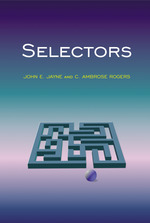# Selectors

John E. Jayne
C. Ambrose Rogers
Pages: 232
https://www.jstor.org/stable/j.ctt7sx8t

1. Front Matter
(pp. i-iv)
(pp. v-vi)
3. Preface
(pp. vii-viii)
J. E. Jayne and C. A. Rogers
4. Introduction
(pp. ix-xiv)

Zermelo  presented a proof that any set can be wellordered. His proof was based on the apparently clear idea that ifMis any set with a given cardinal number, then it is possible for each nonempty subsetM'ofMto choose an elementm'ofM'and call it the distinguished element ofM'.

Borel  wrote accepting that Zermelo had proved that the proposition: “A setMcan be expressed as a wellordered set” was implied by the proposition: “Given an arbitrary nonempty subsetM'of a setMit is possible to choose a point...

5. Chapter 1 Classical results
(pp. 1-18)

Many selection theorems have been proved; many of the earlier ones are called uniformization theorems. One of the important starting points is the work of N. N. Lusin and P. S. Novikov . However, the first general results yielding continuous selectors and selectors of the first Borel class were obtained by E. Michael  and by K. Kuratowski and C. Ryll-Nardzewski . We take these two results as our starting points.

Although Michael’s work was published in three papers in theAnnals of Mathematics[54,55,56], we shall follow his paper in , confining our attention to the special case when...

6. Chapter 2 Functions that are constant on the sets of a disjoint discretely σ-decomposable family of Fσ-sets
(pp. 19-42)

Most of the selectors that we construct in subsequent chapters are obtained as uniform limits of certain “approximate selectors” that are point-valued functions which are constant on the sets of some partition of a space into a disjoint discretely σ-decomposable family of Fσ-sets. Our aim in this chapter is to define and construct such partitions of space and to obtain properties of such functions and their limits. The main conclusions are summarized in Theorem 2.1.

First we need to define one term and to derive some results in the theory of nonseparable metric spaces as developed by Montgomery , Stone...

7. Chapter 3 Selectors for upper semi-continuous functions with nonempty compact values
(pp. 43-64)

In this chapter we obtain selectors of the first Borel class and of the first Baire class for certain upper semi-continuous set-valued functions with nonempty compact values.

Before we state the main results it will be convenient to give some definitions. We suppose thatZis a space with a Hausdorff topologyτand also a metricd, not necessarily related to the topologyτ. We say that(Z,τ)isfragmented down to$\varepsilon \; > 0$byd, if each nonempty subset ofZhas a nonemptyτ-relatively open subset ofd-diameter less than$\varepsilon$. We say that(Z,τ)isfragmented by...

8. Chapter 4 Selectors for compact sets
(pp. 65-90)

In this chapter we derive some results, not dissimilar from those in Chapter 3, from a different point of view. We combine the method of Jayne and Rogers  with methods developed by Ghoussoub, Maurey and Schachermayer  in a paper containing many interesting selection results.

Recall that theHausdorff metric don the space of nonempty bounded closed sets of a metric space$(X,\;\rho )$is defined by

$d(H,{\rm{ }}K) = {\rm{inf\{ }}\varepsilon > 0:H \subset {K_\varepsilon }{\rm{ and }}K \subset {H_\varepsilon }\}$,

where

${H_\varepsilon } = \{ x:\rho (x,{\rm{ }}H) < \varepsilon \}$

is the$\varepsilon {\rm{ - }}neighborhood$of H.

Before we state the main results in this chapter, we state a very simple result that sets the pattern for the subsequent results.

Theorem...

9. Chapter 5 Applications
(pp. 91-122)

In this chapter we mainly use theorems that we have already established to give results for a variety of set-valued maps that have geometric origins. In particular we study subdifferentials, two sorts of attainment maps, metric projections and continuous maps to a space of compact convex sets. For these purposes we study maximal monotone maps. We also give results that act as partial converses to Theorems 3.2 and 3.3.

A set-valued mapFfrom a Banach spaceXto its dual space$X{\kern 1pt} *$is said to be amonotone mapif

$\langle {x_2} - {x_1},x_2^* - x_1^*\rangle \ge 0$

for all choices of${x_1},\;x_1^*,\;{x_2},\;x_2^*$with

$x_1^* \in F({x_1}){\rm{ and }}x_2^* \in F({x_2})$.

Here...

10. Chapter 6 Selectors for upper semi-continuous set-valued maps with nonempty values that are otherwise arbitrary
(pp. 123-146)

In this chapter we prove three main theorems, the first two due to V. V. Srivatsa, and the third by use of his method (see ). Srivatsa obtained his results and wrote them out in detail during a visit to University College London for the session 1984—85; publication was much delayed.

Another account is given by Jayne, Orihuela, Pallarés and Vera .

Theorem 6.1 (Srivatsa)Let X and Y be metric spaces and let F be an upper semi-continuous set-valued map from X to Y taking only nonempty values. Then F has a selector f that is σ-discrete and...

11. Chapter 7 Further applications
(pp. 147-160)

In Chapter 5 we have given some applications of the existence theorems for selectors of the first Baire class, giving detailed proofs based on well known basic results in general topology and Banach space theory. In this chapter we give some further applications, but we only give some of the details of the proofs and have to quote some difficult results without proofs, since the proofs would take us too far from our main themes.

Our results are centered around the theory of Asplund spaces. We start by discussing some of the work done on these spaces.

E. Asplund ...

12. Bibliography
(pp. 161-164)
13. Index
(pp. 165-167)